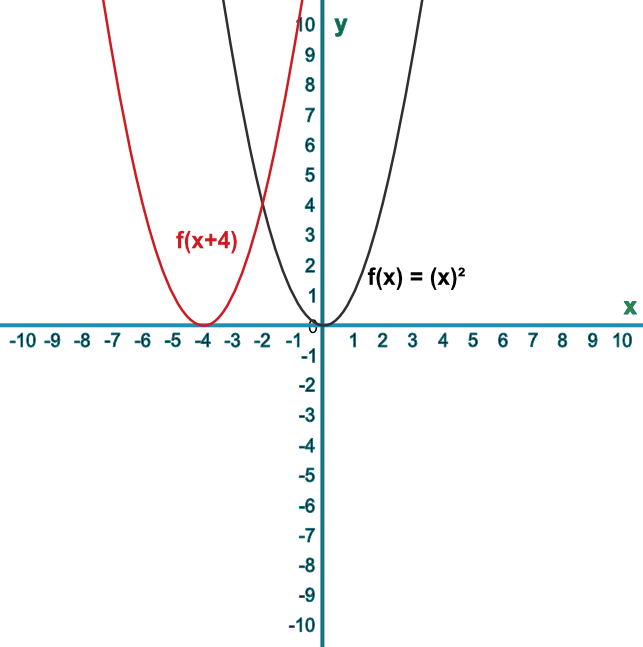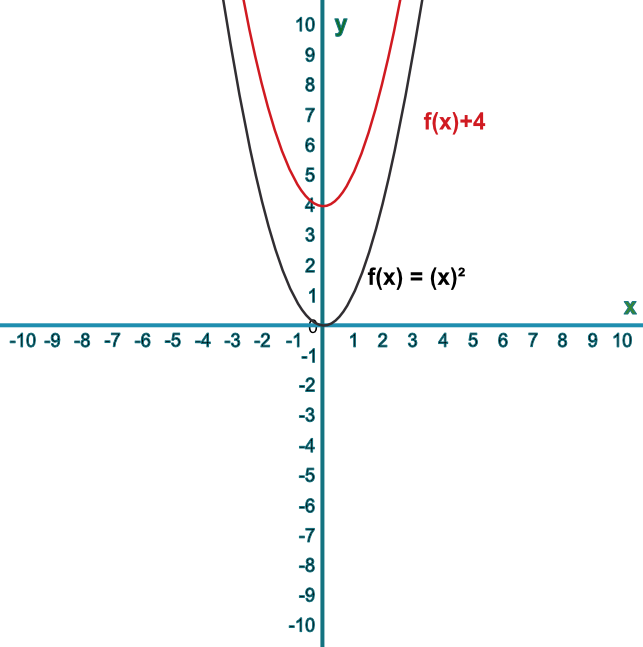Sketch Translations of Functions

## Sketch Translations of Functions

Graphs can be translated horizontally and vertically.

To translate the graph horizontally, add or subtract a value before the function is applied. The movement is in the opposite direction to the value of the addition or subtraction. If y = f(x) then f(x + a) will cause the graph to be translated by ((-a),(0)).

To translate a graph vertically, add or subtract a value after the function is applied. That is, if y=f(x) then y=f(x)+a will result in a translation of ((0),(a)).

## Example 1

Sketch the graph of f(x)=x^2. Then sketch the graph of f(x+4).

Describe the translation.

To translate a function horizontally, apply the add/subtract before evaluating the function.

Answer: The line has been translated ((-4),(0))## Example 2

Sketch the graph of f(x)=x^2. Then sketch the graph of f(x)+4.

Describe the transformation.

To translate a function vertically, apply the add/subtract after evaluating the function.

Answer: The line has been translated ((0),(4))